Home > MC2 > Chapter 11 > Lesson 11.2.1 > Problem11-31

11-31.
1. Imagine a standard deck of cards with all of the aces and twos removed. Find each probability below. Homework Help ✎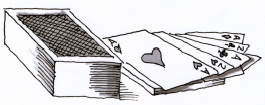1. P(heart)

2. P(black)

3. P(face card)

4. How is the P(face card) different with this deck from the probability if the deck was not missing any cards? Which probability is greater? Why?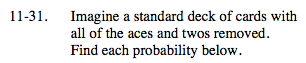How many hearts are there? What is the new total number of cards?

$\frac{11}{44}$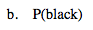How many black cards are there? What is the new total number of cards?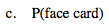What is the number of face cards? What is the new total number of cards?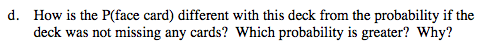What is the probability of drawing a face card when the deck is not missing any cards?

Compare this probability with the one found in (c).

The probability of drawing a face card with aces and twos removed is higher because there are fewer non-face cards.